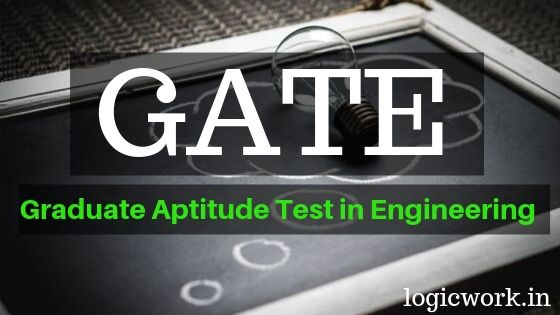# Gate latest syllabus for Computer Science and Information Technology (CS)

### GATE 2020 Syllabus and Paper Pattern

 GATE Paper (Code) Computer Science and Information Technology (CS) Total Number of Questions 65 Total Marks 100 Time Duration of Exam 3 Hours Marks Distribution 15 Marks from General Aptitude15 Marks from Engineering Mathematics70 Marks from Core Subjects of Paper

#### 1. General Aptitude

Verbal Ability: English grammar, sentence completion, verbal analogies, word groups, instructions, critical reasoning and verbal deduction.
Numerical Ability: Numerical computation, numerical estimation, numerical reasoning and data interpretation.

#### 2. Engineering Mathematics

Discrete Mathematics: Propositional and first order logic. Sets, relations, functions, partial orders and lattices. Groups. Graphs: connectivity, matching, coloring. Combinatorics: counting, recurrence relations, generating functions.

Linear Algebra: Matrices, determinants, system of linear equations, eigenvalues and eigenvectors, LU decomposition.

Calculus: Limits, continuity and differentiability. Maxima and minima. Mean value theorem. Integration.

Probability: Random variables. Uniform, normal, exponential, poisson and binomial distributions. Mean, median, mode and standard deviation. Conditional probability and Bayes theorem.

#### 3. Digital Logic

Boolean algebra. Combinational and sequential circuits. Minimization. Number representations and computer arithmetic (fixed and floating point).

#### 4. Computer Organization and Architecture

Machine instructions and addressing modes. ALU, data-path and control unit. Instruction pipelining. Memory hierarchy: cache, main memory and secondary storage; I/O interface (interrupt and DMA mode).

#### 5. Programming and Data Structures

Programming in C. Recursion. Arrays, stacks, queues, linked lists, trees, binary search trees, binary heaps, graphs.

#### 6. Algorithms

Searching, sorting, hashing. Asymptotic worst case time and space complexity. Algorithm design techniques: greedy, dynamic programming and divide-and-conquer. Graph search, minimum spanning trees, shortest paths.

#### 7. Theory of Computation

Regular expressions and finite automata. Context-free grammars and push-down automata. Regular and contex-free languages, pumping lemma. Turing machines and undecidability.

#### 8. Compiler Design

Lexical analysis, parsing, syntax-directed translation. Runtime environments. Intermediate code generation.

#### 9. Operating System

Processes, threads, inter-process communication, concurrency and synchronization. Deadlock. CPU scheduling. Memory management and virtual memory. File systems.

#### 10. Databases

ER-model. Relational model: relational algebra, tuple calculus, SQL. Integrity constraints, normal forms. File organization, indexing (e.g., B and B+ trees). Transactions and concurrency control.

#### 11. Computer Networks

Concept of layering. LAN technologies (Ethernet). Flow and error control techniques, switching. IPv4/IPv6, routers and routing algorithms (distance vector, link state). TCP/UDP and sockets, congestion control. Application layer protocols (DNS, SMTP, POP, FTP, HTTP). Basics of Wi-Fi. Network security: authentication, basics of public key and private key cryptography, digital signatures and certificates, firewalls.